# Measuring the phase noise of a laser

2021-07-08

In this post, we present a setup to measure the phase noise of narrow linewidth laser diodes using a self-heterodyne Mach-Zehnder interferometer with short delay. With this method, we can track the laser phase and compute the laser phase noise density. This provides a detailed analysis of the laser frequency stability, in particular for narrow linewidth lasers with non-Lorentzian lineshapes.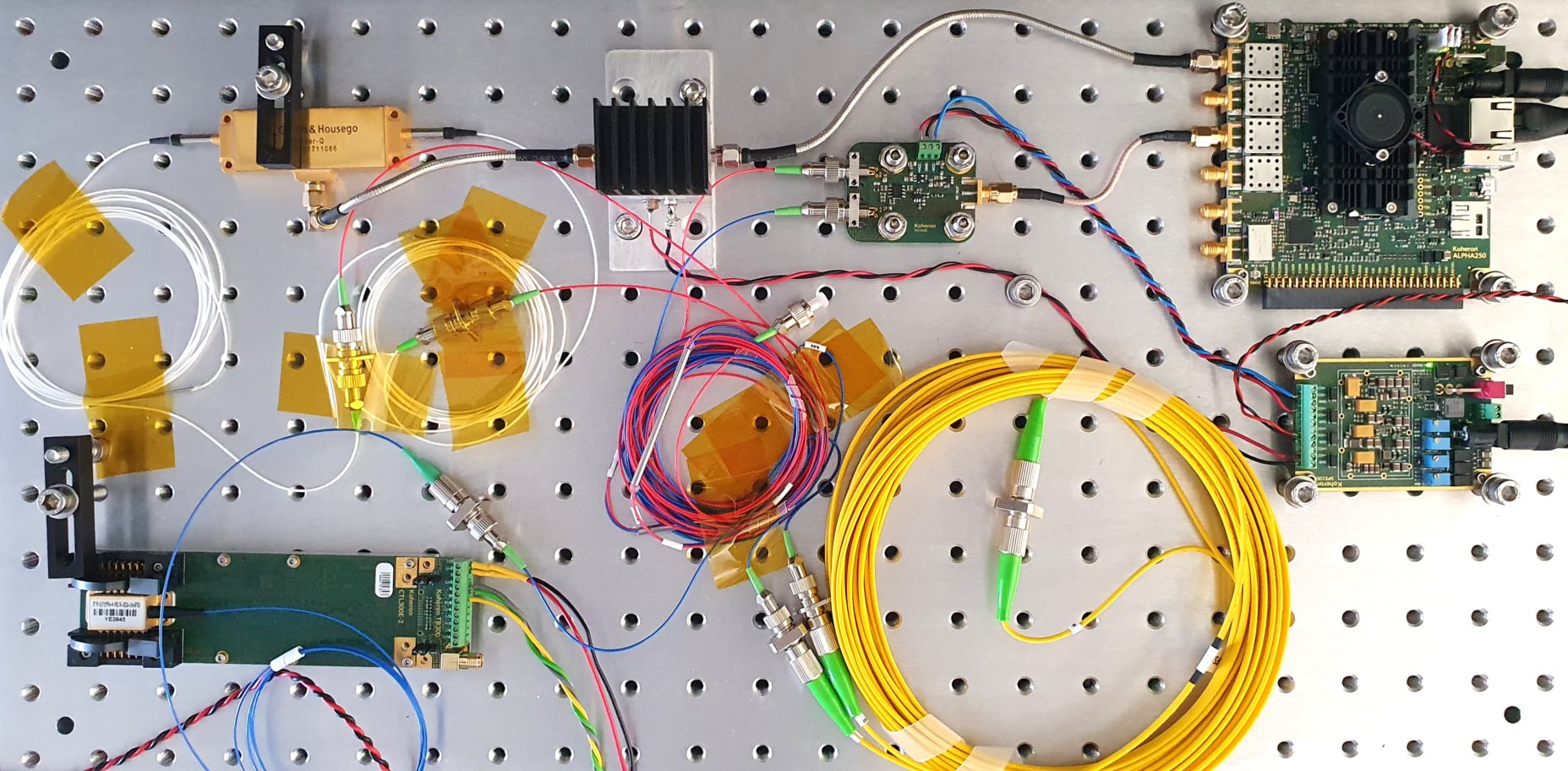## Experimental setup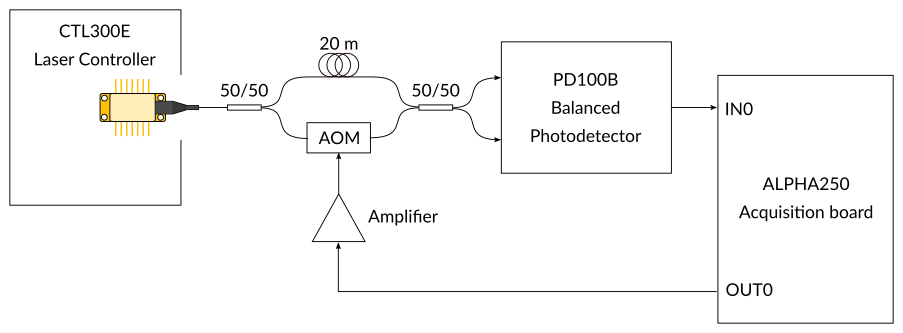The output of a laser controlled by a CTL300E laser controller feeds a Mach-Zehnder interferometer built with two 50/50 fiber splitters. On one arm, the laser wave is delayed by a 20 m long fiber. On the other arm, a fibered acousto-optic modulator (AOM) shifts the laser frequency by 40 MHz. A PD100B-DC balanced photodetector converts the interferometer output into an electrical signal which is then digitized on an ALPHA250 acquisition board. A single SPS100-HV power supply powers the whole system.

## From interferometric signal to laser phase noise

Let $\varphi_L(t)$ be the laser phase, $\omega_{s} / 2 \pi$ the AOM modulation frequency and $\tau$ the time delay between the 2 interferometer arms. The intensity at the interformeter output is then (omitting constant phases):

$$I = I_{0} \left\{ 1 + \cos \left[ \omega_{s} t - \left( \varphi_L(t + \tau) - \varphi_L(t) \right) \right] \right\}$$

After demodulation by the carrier at $\omega_{s}$, the phase noise analyzer computes the phase noise of the RF signal $\phi_{\mathrm{RF}}(t) = \varphi_L(t + \tau) - \varphi_L(t)$, whose Fourier transform is $\hat{\phi}_{\mathrm{RF}} (\omega) = \hat{\varphi}_{L} (\omega) \left( e^{- i \omega \tau} - 1 \right)$. The relationship between the laser phase noise and the RF phase noise is thus:

$$S_{\varphi_L} (\omega) \equiv \left| \hat{\varphi}_{L} (\omega) \right|^{2} = S_{\phi_{\mathrm{RF}}} (\omega) \left| e^{- i \omega \tau} - 1\right|^{-2} = \frac{S_{\phi_{\mathrm{RF}}} (\omega)}{ 4 \sin^{2} \left( \omega \tau / 2 \right)},$$

where $S_{\varphi} (\omega)$ is the phase noise power spectral density of $\varphi$ (in rad2/Hz).

In what follows we plot the frequency noise power spectral density (in Hz2/Hz):

$$S_{\nu} (f) = f^{2} S_{\varphi} (f).$$

## Interferometer delay calibration

Knowledge of the interferometer delay is necessary to convert the RF phase noise into the laser phase noise.

Here is the power spectrum of the photodetector output centered at 40 MHz, measured using the FFT Analyzer instrument: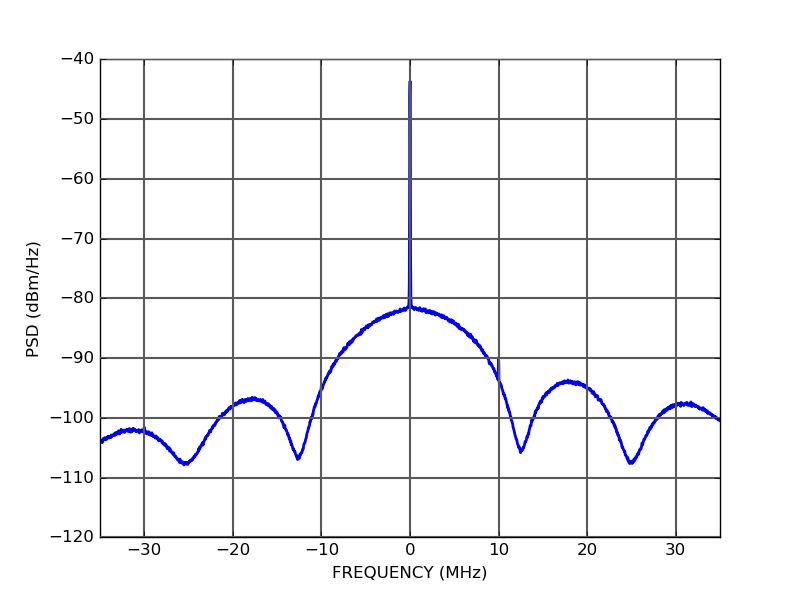We observe a sinc-squared-like spectrum whose zeros are related to the interferometric delay. Here the first zero is located at an offset $f_0$ = 12.6 MHz from the carrier. The delay is thus $\tau = 1 / f_0$ = 79.3 ns (that is a delay length $L = c \tau / n$ = 16.4 m, for $n$ = 1.45).

## Digital signal processing

The interferometric signal is processed by the Phase Noise Analyzer instrument running on the ALPHA250 acquisition board: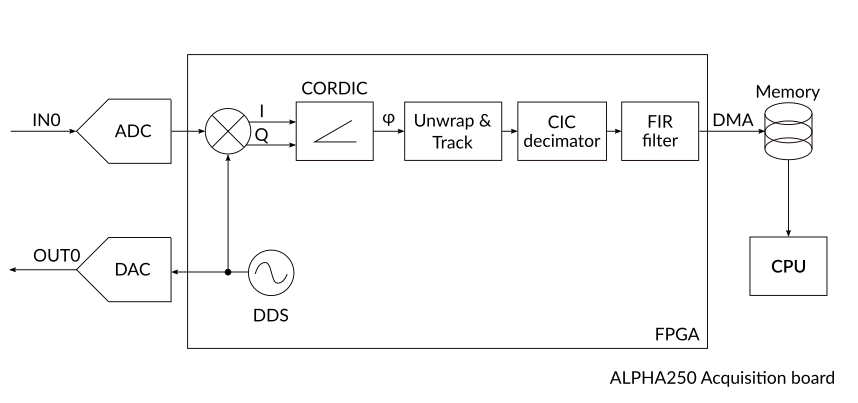A Direct Digital Synthesizer (DDS) generates the 40 MHz carrier frequency. It is converted to an analog signal to drive the AOM and used to demodulate the digitized photodetector output signal.

A COordinate Rotation DIgital Computer (CORDIC) is used to extract the phase of the demodulated signal. The phase is then unwrapped and accumulated, providing full tracking without phase jumps.

The phase is then decimated, filtered and stored in memory before being transferred to a host computer where a Python script computes the phase noise power spectral density.

## Some measurements

The figure below shows the frequency noise of an Eblana EP1550-0-NLW-B26-100FM DFB laser driven at 180 mA with a CTL200-2-B-200 laser controller and a CTL300E-2-400 laser controller. The dotted lines correspond to the white frequency noise of ideal Lorentzian lasers with linewidths of 10 kHz, 100 kHz and 1 MHz.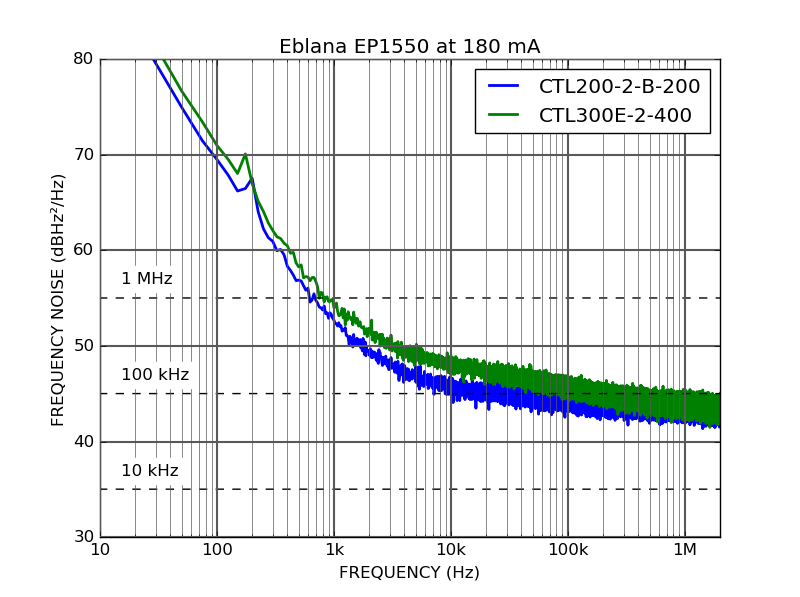When driven with the CTL300E-2-400 laser controller, the laser has an excess frequency noise between 1 kHz and 100 kHz. This is explained by the fact that the current noise density of the CTL300E-2-400 laser controller is about 800 pA/√Hz at 10 kHz (vs. 220 pA/√Hz for the CTL200-2-B-200 laser controller). At 1 MHz, the frequency noise is no longer limited by the current noise but by the laser itself.

The figure below shows the frequency noise of an Eblana EP1550-0-NLW-B26-100FM DFB laser driven at 180 mA with a CTL101-2-B-200 laser controller and of a Thorlabs SFL1550P external cavity laser driven at 200 mA with a CTL101-1-B-400 laser controller.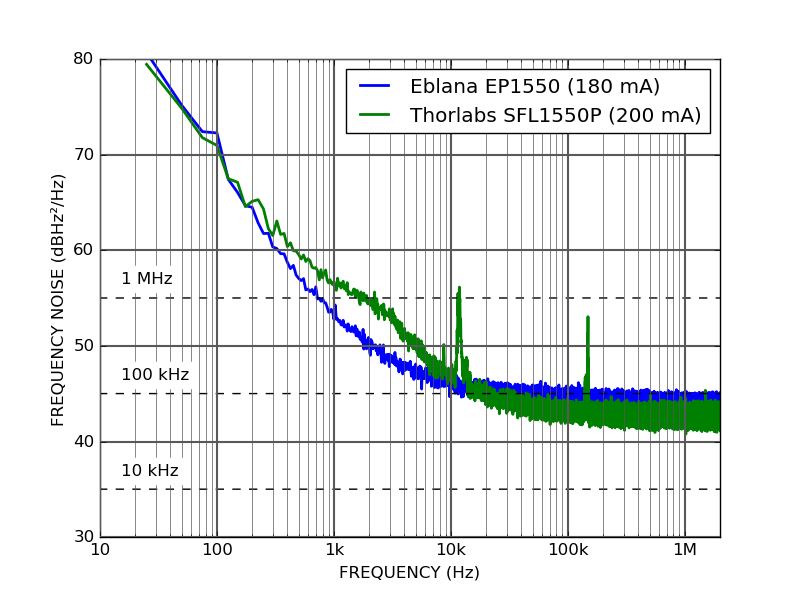## Python code

Here is the Python script used to compute the laser phase noise with the Phase Noise Analyzer instrument running on the ALPHA250 acquisition board.

import numpy as np
import matplotlib
from matplotlib import pyplot as plt
import os
import time
from koheron import connect, command

class PhaseNoiseAnalyzer(object):
def __init__(self, client):
self.client = client

@command(classname="Dds")
def set_dds_freq(self, channel, freq):
pass

@command()
def get_data(self):
return self.client.recv_array(200000, dtype='int32')

freq = 40E6   # Modulation frequency (Hz)
tau = 79.3E-9 # Interferometer delay (s)

host = os.getenv('HOST','192.168.1.114')
driver = PhaseNoiseAnalyzer(connect(host, 'phase-noise-analyzer'))
driver.set_dds_freq(0, freq)

n = 200000
cic_rate = 20
fs = 200E6 / (cic_rate * 2)
n_avg = 100

f = np.arange((n/2 + 1)) * fs / n
window = np.ones(n)
psd = np.zeros(n/2 + 1)

for i in range(n_avg):
print("Acquiring sample {}/{}".format(i + 1, n_avg))
data = driver.get_data()
data = data - np.mean(data)
calib_factor = 4.196
data *= calib_factor * np.pi / 8192.0 # rad
psd += 2.0 * np.abs(np.fft.rfft(window * data))**2

psd /= n_avg
psd /= (fs * np.sum(window ** 2)) # rad^2/Hz
psd_dB = 10.0 * np.log10(psd / 2.0) # Convert to dBc/Hz
psd_dB -= 20.0 * np.log10(2.0 * np.sin(np.pi * f * tau)) # Interferometer transfer function

ax = plt.subplot(111)
ax.semilogx(f, psd_dB, linewidth=2)
ax.set_xlabel("FREQUENCY (Hz)")
ax.set_ylabel("SSB PHASE NOISE (dBc/Hz)")
ax.grid(True, which='major', linestyle='-', linewidth=1.5, color='0.35')
ax.grid(True, which='minor', linestyle='-', color='0.35')
plt.show()